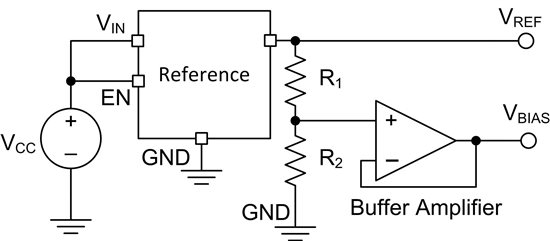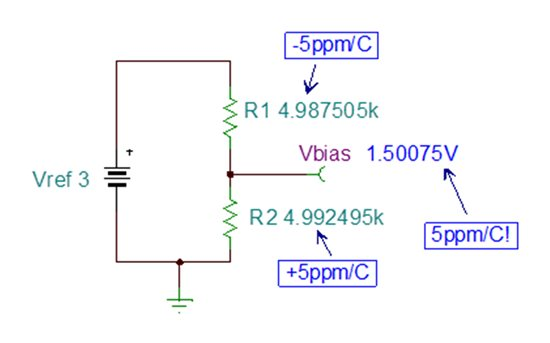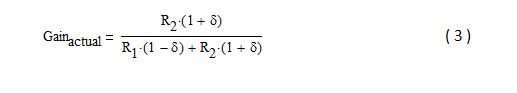If you have a voltage divider where each resistor has 5 ppm/°C drift, what is the worst-case drift?  That is the loaded question I posed to my colleagues recently (only after figuring out the answer myself, of course) while working on a low-drift current sensing reference design (TIPD156).  The ‘obvious’ answer is 10 ppm/°C.  It turns out to be just 5 ppm/°C, but only when the voltage divider ratio is ½.  Let us delve deeper into the answer to this obvious, yet not-so-obvious question.

Figure 1 depicts a discrete solution that provides a reference voltage (VREF) and bias voltage (VBIAS) based on the ratio of R1 and R2.Figure 1: Dual reference discrete topology

At the time it was ‘obvious’ that the overall drift of the resistor divider is (5 ppm/°C) + (5 ppm/°C) = 10 ppm/°C.  Just to be sure, though, I ran a simulation.  Figure 2 depicts the result of the TINA-TI simulation where R2 drifts in the positive direction, R1 drifts negatively, and the change in temperature is 100°C.Figure 2: Vbias after R1 and R2 drift

Calculating the gain error yields 0.05% (500 ppm or 5.0 ppm/°C), which is the drift of one resistor…not their sum!

Now let’s examine the familiar voltage divider circuit’s gain ratio and re-arrange it as shown in Equation ( 1 ).

α is the ratio of R1 and R2, and ultimately relates to the gain of the circuit.  For example, if α=1, the gain is ½.  So, as α→0, the gain→1.  Similarly, as α→∞, gain→ 0.

The actual gain of the circuit over temperature will depend on the drift of the resistors, which is shown in Equation ( 3 ) by δ.  To be consistent with the simulation, R2 drifts positively and R1 negatively.Equation ( 4 ) calculates the gain error.  For simplicity, it is not converted to a percentage (if you want to do that, simply multiply it by 100 but be careful when converting to ppm).Using Equations ( 1 ) to ( 3 ), Equation ( 4 ) simplifies to:

Notice that the gain error depends on both the drift (δ) and the circuit gain (related to α).  Furthermore, if α=1 (circuit gain=½), the gain error simplifies to δ, which is exactly what the simulation shows!

Figure 3 is a plot of the gain error versus the circuit gain for this design (5 ppm/°C resistor drift, or 500 ppm total drift over 100°C).  Notice that the gain error is 500 ppm when the circuit gain is ½.  Also notice that as the circuit gain increases, the gain error decreases and vice versa.

In summary, the drift of the voltage divider was not as expected…it is not the simple sum and it depends on the circuit gain.  So, next time you’re designing a circuit and come across one of those ‘obvious’ conclusions, you may want to double-check it with TINA-TI!

If you have any similar ‘obvious’ experiences, please share them in the comments section!

• Lothar,

When I compare the results of your equation to the results of Equation 5 there appears to be a fixed error in the results related to the TK term used. I will try to describe my findings by example. If TK (or drift) is 100ppm (.0001) then the result of your equation has a fixed .0001x difference when compared to the results of Equation 5. If TK is 50ppm the difference between the results is 0.00005x.

It is possible I am not interpreting "TK" or "division ratio" correctly. I assumed if R1=1000 and R2=1 then "division ratio"=1000.

The result is very close and a good approximation with a much simpler equation, just not exact. Please check my results and let me know if I'm wrong - it won't be the first time.

For what it's worth the brute force method I used in Excel agrees with the results of Equation 5 to what I assume is round-off error in Excel: less than +/- 3x10^(-16).

MKARGER,

The articles discussion of drift varying with time relates to aging calculations which are part of reliability calculations which can be performed on all components. The "law of Arrhenius" tends to be tailored to the component being evaluated.

• MKARGER - Without reading the Vishay application note (problem with Adobe I need to resolve) I know they make some precision devices and I do not doubt if they say the "drift" changes with duration a load is applied - they should know. Thanks for the link I look forward to reading it. My understanding is that in most film resistors the pastes used for screening the resistors are modified to result in as close to zero tempco as is merited for the part. But getting close could still result in either a positive or a negative tempco in which case the values of any two parts can still diverge from each other as temperature changes.

• Interesting read of an often-overlooked topic!  However, is it valid to assume that one resistor can drift positive while the other drifts negative?  Since you're talking about a voltage divider, the resistors are presumably in close proximity to each otehr, and therefore see roughly the same ambient temperature.  I was under the impression that resistors drifted up for increasing ambient temperature, and down for decreasing temperature.  So they should drift up or down together, no?

This tech note from Vishay also highlights that the drift of a thin film resistor isn't a constant, but that it increases with the amount of time the load is applied:  www.vishay.com/.../driftcalculation.pdf

• It is an interesting observation that I also overlooked for many years until I used Excel to graph the effect of any tolerance artifact for a voltage divider. The ratio and location of the two resistors in the divider defines the extent of the error that can be expected. Assuming both resistors have the same tolerance, when the resistors are of equal value the maximum error is equal to the tolerance not the sum of tolerances. When, in a simple divider, the low-side resistor is significantly less than the high-side resistor the resulting error approaches the sum of the tolerances. Reverse the relative locations and the error approaches zero. Plotting the range over 0.001 to 1000 on a log scale is a great way to visualize the point.

• Hallo,

there seem to be a simple context when I look at figure 3. This formula is easyer to remeber:

Gain error = 2 x TK x (1 - division ratio) x delta T

(delta T is the changn in temperatur)  Is the formula right or are there any argument against it?

Regards from Berlin